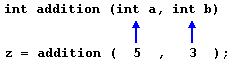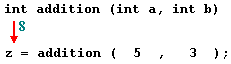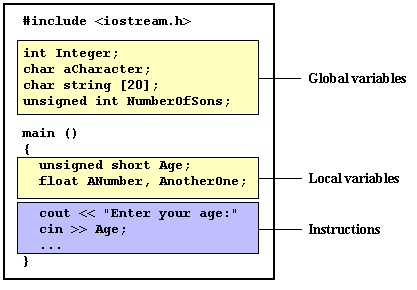# C++ 函数

type name ( argument1, argument2, ...) statement

• type 是函数返回的数据的类型
• name 是函数被调用时使用的名
• argument 是函数调用需要传入的参量(可以声明任意多个参量)。每个参量(argument)由一个数据类型后面跟一个标识名称组成，就像变量声明中一样(例如，int x)。参量仅在函数范围内有效，可以和函数中的其它变量一样使用， 它们使得函数在被调用时可以传入参数，不同的参数用逗号(comma)隔开.
• statement 是函数的内容。它可以是一句指令，也可以是一组指令组成的语句块。如果是一组指令，则语句块必须用花括号{}括起来，这也是我们最常见到情况。其实为了使程序的格式更加统一清晰，建议在仅有一条指令的时候也使用花括号，这是一个良好的编程习惯。

// function example
#include <iostream.h>
int addition (int a, int b)
{
int r;
r=a+b;
return (r);
}

int main ()
{
int z;
cout << "The result is " << z;
return 0;
}

The result is 8return (r);cout << "The result is " << z;变量的范围(Scope of variables)

// function example
#include <iostream.h>
int subtraction (int a, int b)
{
int r;
r=a-b;
return (r);
}

int main ()
{
int x=5, y=3, z;
z = subtraction (7,2);
cout << "The first result is " << z << '\n';
cout << "The second result is " << subtraction (7,2) << '\n';
cout << "The third result is " << subtraction (x,y) << '\n';
z= 4 + subtraction (x,y);
cout << "The fourth result is " << z << '\n';
return 0;
}

The first result is 5
The second result is 5
The third result is 2
The fourth result is 6

z = subtraction (7,2);
cout << "The first result is " << z;

z = 5;
cout << "The first result is " << z;

cout << "The second result is " << subtraction (7,2);

cout << "The second result is " << 5;

cout << "The third result is " << subtraction (x,y);

z = 4 + subtraction (x,y);

z = subtraction (x,y) + 4;

z = 4 + 2;
z = 2 + 4;

## 没有返回值类型的函数，使用void.

type name ( argument1, argument2 ...) statement

`// void 函数示例#include <iostream>using namespace std;void printmessage (){  cout << "I'm a function!";}int main (){  printmessage ();  return 0;}`

I'm a function!

void还可以被用在函数参数位置，表示我们明确希望这个函数在被调用时需要任何参数。例如上面的函数printmessage也可以写为以下形式：

`void printmessage (void){  cout << "I'm a function!";}`

printmessage();

printmessage;

## 参数按数值传递和按地址传递(Arguments passed by value and by reference)

int x=5, y=3, z;
z = addition ( x , y );// passing parameters by reference
#include <iostream.h>

void duplicate (int& a, int& b, int& c)
{
a*=2;
b*=2;
c*=2;
}

int main ()
{
int x=1, y=3, z=7;
duplicate (x, y, z);
cout << "x=" << x << ", y=" << y << ", z=" << z;
return 0;
}

x=2, y=6, z=14void duplicate (int& a, int& b, int& c)

void duplicate (int a, int b, int c)

// more than one returning value
#include <iostream.h>
void prevnext (int x, int& prev, int& next)
{
prev = x-1;
next = x+1;
}

int main ()
{
int x=100, y, z;
prevnext (x, y, z);
cout << "Previous=" << y << ", Next=" << z;
return 0;
}

Previous=99, Next=101

## 参数的默认值(Default values in arguments)

// default values in functions
#include <iostream.h>
int divide (int a, int b=2) {
int r;
r=a/b;
return (r);
}

int main () {
cout << divide (12);
cout << endl;
cout << divide (20,4);
return 0;
}

6
5

divide (12)

divide (20,4)

#include <iostream.h>

int divide (int a, int b) {
return (a/b);
}

float divide (float a, float b) {
return (a/b);
}

int main () {
int x=5,y=2;
float n=5.0,m=2.0;
cout << divide (x,y);
cout << "\n";
cout << divide (n,m);
cout << "\n";
return 0;
}

2
2.5

## Inline 函数（inline functions）

inline type name ( arguments ... ) { instructions ... }

## 递归（Recursivity）

n! = n * (n-1) * (n-2) * (n-3) ... * 1

5! = 5 * 4 * 3 * 2 * 1 = 120

// factorial calculator
#include <iostream.h>

long factorial (long a){
if (a > 1) return (a * factorial (a-1));
else return (1);
}

int main () {
long l;
cout << "Type a number: ";
cin >> l;
cout << "!" << l << " = " << factorial (l);
return 0;
}

Type a number: 9
!9 = 362880

## 函数的声明（Declaring functions）

type name ( argument_type1, argument_type2, ...);

• 它不包括函数的内容， 也就是它不包括函数后面花括号{}内的所有语句。
• 它以一个分号semicolon sign (;) 结束。
• 在参数列举中只需要写出各个参数的数据类型就够了，至于每个参数的名字可以写，也可以不写，但是我们建议写上。

// 声明函数原型
#include <iostream.h>

void odd (int a);
void even (int a);

int main () {
int i;
do {
cout << "Type a number: (0 to exit)";
cin >> i;
odd (i);
} while (i!=0);
return 0;
}

void odd (int a) {
if ((a%2)!=0) cout << "Number is odd.\n";
else even (a);
}

void even (int a) {
if ((a%2)==0) cout << "Number is even.\n";
else odd (a);
}

Type a number (0 to exit): 9
Number is odd.
Type a number (0 to exit): 6
Number is even.
Type a number (0 to exit): 1030
Number is even.
Type a number (0 to exit): 0
Number is even.

void odd (int a);
void even (int a);